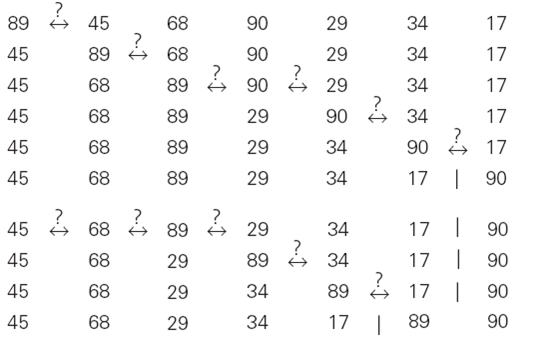# Bubble Sort Program In C

## BUBBLE SORT IN C

Back To Bubble Sort

Bubble Sort is a popular but inefficient sorting algorithm.  Bubble Sort is another brute-force application to the sorting problem that compares adjacent elements of the list and exchange them if they are out of order. By doing it repeatedly, we end up "bubbling up" the largest element to the last position on the list. The next pass bubbles up the second largest element, and so on until, after n - 1 passes, the list is sorted. Pass i (0 <= i <= n - 2) of bubble sort can be represented by the following diagram:

Consider element to be sorted are : 89, 45, 68, 90, 29, 34, 17Quick Sort In C : Showing how elements are sorted

First two passes of bubble sort on the given list 89, 45, 68, 90, 29, 34, 17 is shown in above figure.  A new line is shown after a swap of two elements is done. The elements to the right of the vertical bar are in their final positions and are not considered in  subsequent iterations of the algorithm.

## BUBBLE SORT ALGORITHM

`// Algorithm for Bubble Sort`
`BubbleSort(int a[],int n)`
```  for i=0 to n-2
```
`    for j=0 to n-2-i`
`      if a[j] > a[j+1]`
`        swap a[j] with a[j+1]`

## BUBBLE SORT PROGRAM IN C

```// Bubble sort program in c

#include<stdio.h>

void bubblesort(int a[],int n);

int main()
{
int a,i,n;
printf("Enter number of elements in array : ");
scanf("%d",&n);

// Inputting elements
for(i=0;i<n;i++)
{
printf("Enter number %d: ",i+1);
scanf("%d",&a[i]);
}

// Displaying Elements before bubble sort
printf("Items in the array are : ");
for(i=0;i<n;i++)
{
printf("%d ",a[i]);
}

//Applying bubble sort
bubblesort(a,n);

// Displaying elements after bubble sort
printf("\nElements after bubble sort : ");
for(i=0;i<n;i++)
{
printf("%d ",a[i]);
}
return 0;
}

void bubblesort(int a[],int n)
{
int i,j;
for(i=0; i<=n-2; i++)
{
for(j=0;j<=n-i-2;j++)
{
// Swapping elements
if(a[j]>a[j+1])
{
int temp=a[j];
a[j]=a[j+1];
a[j+1]=temp;
}
}
}
}

```